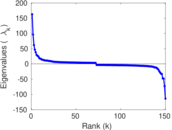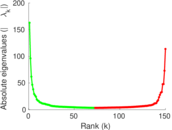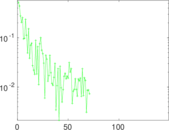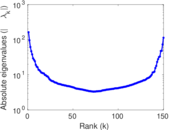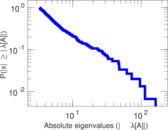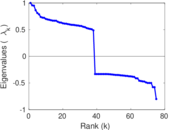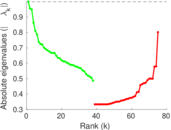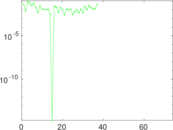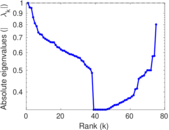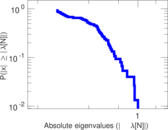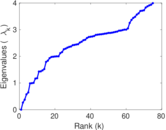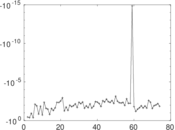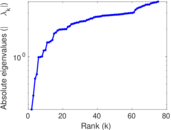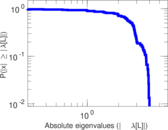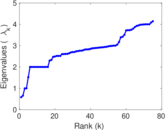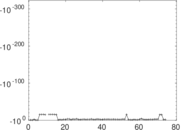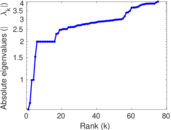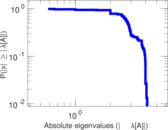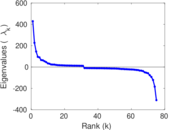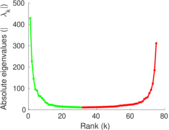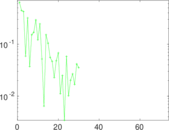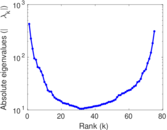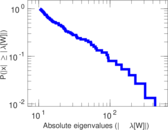Caenorhabditis elegans

This is the metabolic network of the roundworm Caenorhabditis elegans. Nodes are metabolites (e.g., proteins), and edges are interactions between them. Since a metabolite can iteract with itself, the network contains loops. The interactions are undirected. There may be multiple interactions between any two metabolites.

 Code PM Internal name arenas-meta Name Caenorhabditis elegans Data source http://deim.urv.cat/~aarenas/data/welcome.htm AvailabilityDataset is available for download Consistency checkDataset passed all tests Category Metabolic network Node meaning Metabolite Edge meaning Interaction Network format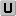Unipartite, undirected Edge typeUnweighted, multiple edges Loops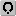Contains loops

Statistics

 Size n = 453 Volume m = 4,596 Unique edge count m̿ = 2,040 Loop count l = 22 Wedge count s = 79,173 Claw count z = 3,352,172 Cross count x = 153,983,040 Triangle count t = 3,284 Square count q = 50,289 4-Tour count T4 = 723,054 Maximum degree dmax = 639 Average degree d = 20.291 4 Fill p = 0.019 838 4 Average edge multiplicity m̃ = 2.252 94 Size of LCC N = 453 Diameter δ = 7 50-Percentile effective diameter δ0.5 = 2.081 97 90-Percentile effective diameter δ0.9 = 3.033 51 Median distance δM = 3 Mean distance δm = 2.641 74 Gini coefficient G = 0.619 161 Balanced inequality ratio P = 0.268 712 Relative edge distribution entropy Her = 0.898 868 Power law exponent γ = 1.563 30 Tail power law exponent γt = 2.621 00 Tail power law exponent with p γ3 = 2.621 00 p-value p = 0.125 000 Degree assortativity ρ = −0.225 821 Degree assortativity p-value pρ = 5.419 17 × 10−48 Clustering coefficient c = 0.124 436 Spectral norm α = 162.930 Algebraic connectivity a = 0.264 802 Spectral separation |λ1[A] / λ2[A]| = 1.432 48 Non-bipartivity bA = 0.301 910 Normalized non-bipartivity bN = 0.200 552 Algebraic non-bipartivity χ = 0.293 559 Spectral bipartite frustration bK = 0.008 148 41 Controllability C = 8 Relative controllability Cr = 0.017 660 0

Plots

Fruchterman–Reingold graph drawing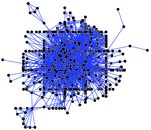Degree distribution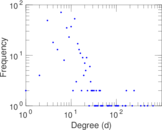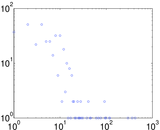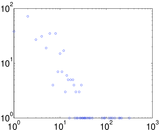Cumulative degree distribution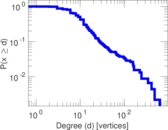Lorenz curve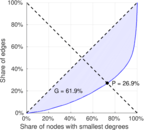Spectral distribution of the adjacency matrix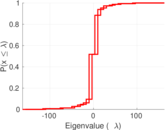Spectral distribution of the normalized adjacency matrix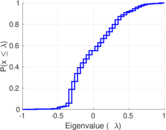Spectral distribution of the Laplacian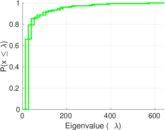Spectral graph drawing based on the adjacency matrix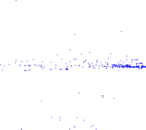Spectral graph drawing based on the Laplacian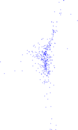Spectral graph drawing based on the normalized adjacency matrix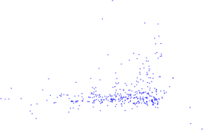Degree assortativity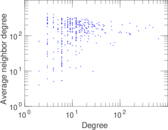Zipf plot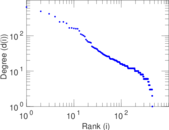Hop distribution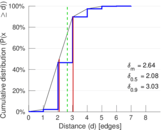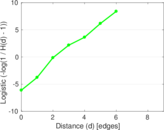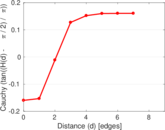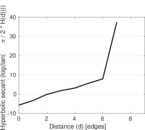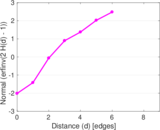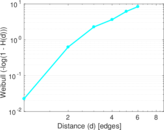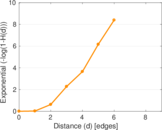Double Laplacian graph drawing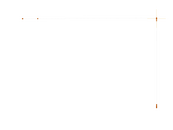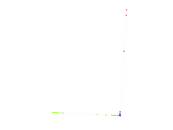Delaunay graph drawing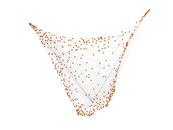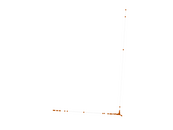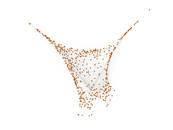Edge weight/multiplicity distribution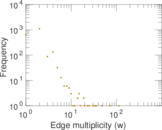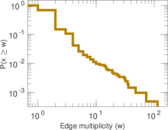Clustering coefficient distribution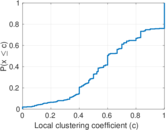Average neighbor degree distribution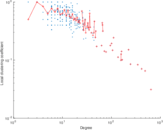SynGraphy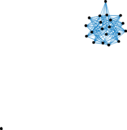Matrix decompositions plots